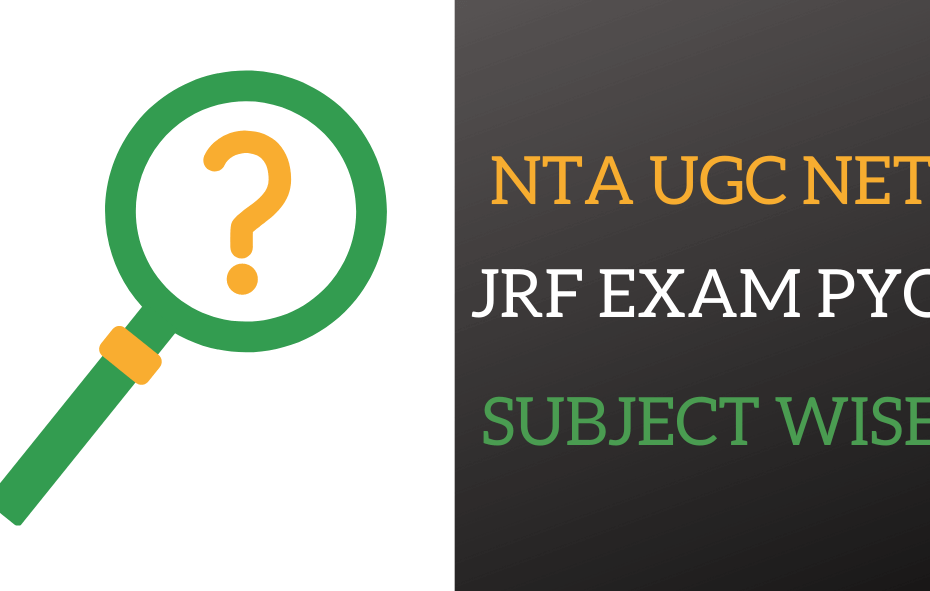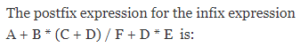# NTA UGC NET Computer Science PYQ Subject Wise

• PYQHere we published NTA UGC NET or GATE Computer Science PYQ Subject Wise only. If you want to solve NTA UGC NET or GATE Previous Year Question Year wise click here.

DATA COMMUNICATION AND COMPUTER NETWORKS

NTA UGC NET Computer Network

1 / 15

Category: Data Communication and Computer Networks

Suppose that everyone in a group of N people wants to communicate secretly with (N-1) other people using symmetric key cryptographic system. The communication between any two persons should not be decodable by the others in the group. The number of keys required in the system as a whole to satisfy the confidentiality requirement is

2 / 15

Category: Computer Networks

Consider an IP packet with a length of 4,500 bytes that includes a 20-byte IPv4 header and a 40-byte TCP header. The packet is forwarded to an IPv4 router that supports a Maximum Transmission Unit (MTU) of 600 bytes. Assume that the length of the IP header in all the outgoing fragments of this packet is 20 bytes. Assume that the fragmentation offset value stored in the first fragment is 0.

The fragmentation offset value stored in the third fragment is __________.

3 / 15

Category: Computer Networks

Match the following:

Field Length in bits
P. UDP Header's Port Number                                        I. 48
Q. Ethernet MAC Address                                              II. 8
S. TCP Header's Sequence Number                                IV. 16

4 / 15

Category: Data Communication and Computer Networks

The IP address ................... is used by hosts when they are being booted.

5 / 15

Category: Computer Networks

A network has a data transmission bandwidth of 20 × 106 bits per second. It uses CSMA/CD in the MAC layer. The maximum signal propagation time from one node to another node is 40 microseconds. The minimum size of a frame in the network is _________ bytes.

6 / 15

Category: Computer Networks

Which one of the following is not typically provided by source code management software

7 / 15

Category: Data Communication and Computer Networks

Which of the following devices takes data sent from one network device and forwards it to
the destination node based on MAC address?

8 / 15

Category: Data Communication and Computer Networks

Which of the following is/are example(s) of stateful application layer protocols?

9 / 15

Category: Computer Networks

A computer network uses polynomials over GF(2) for error checking with 8 bits as information bits and uses x3 + x + 1 as the generator polynomial to generate the check bits. In this network, the message 01011011 is transmitted as

10 / 15

Category: Computer Networks

What is the data unit of physical layer

11 / 15

Category: Data Communication and Computer Networks

1) The address of a class B host is to be split into subnets with a 6-bit subnet number. What is the maximum number of subnets and the maximum number of hosts in each subnet?

12 / 15

Category: Data Communication and Computer Networks

.................. do not take their decisions on measurements or estimates of the current traffic
and topology.

13 / 15

Category: Data Communication and Computer Networks

Which of the following statements is/are true?

14 / 15

Category: Computer Networks

Repeater operates in which layer?

15 / 15

Category: Computer Networks

Calculate Network ID

0%

0%

Data Structures and AlgorithmNTA UGC NET - Data Structures and Algorithms

In this quiz, we focused on NTA UGC NET exam question or GATE question which is related to Data Structures and Algorithms.

1 / 6

Category: Data structure and algorithms

Let T(n) be a function defined by the recurrence T(n) = 2T(n/2) + √n for n ≥ 2 and T(1) = 1 Which of the following statements is TRUE?

2 / 6

Category: Data structure and algorithms3 / 6

Category: Data structure and algorithms

What is the worst case time complexity of inserting n2 elements into an AVL-tree with n elements initially?

4 / 6

Category: Data structure and algorithms

The preorder traversal sequence of a binary search tree is 30, 20, 10, 15, 25, 23, 39, 35, 42. Which one of the following is the postorder traversal sequence of the same tree?

5 / 6

Category: Data structure and algorithms

Let T be a tree with 10 vertices. The sum of the degree of all the vertices in T is

6 / 6

Category: Data structure and algorithms

Which of the following statements is/are TRUE for undirected graphs?
P: Number of odd degree vertices is even.
Q: Sum of degrees of all vertices is even.

0%
0%

Data Structures and Algorithm

Data Structures and Algorithms

In this quiz, we focused on NTA UGC NET exam question or GATE question which is related to Data Structures and Algorithms.

1 / 8

Category: Data structure and algorithms

The preorder traversal of a binary search tree is 15, 10, 12, 11, 20, 18, 16, 19.
Which one of the following is the postorder traversal of the tree?

2 / 8

Category: Data structure and algorithms

Let T(n) be a function defined by the recurrence T(n) = 2T(n/2) + √n for n ≥ 2 and T(1) = 1 Which of the following statements is TRUE?

3 / 8

Category: Data structure and algorithms

The second smallest of n elements can be found with _______ comparisons in the worst case.

4 / 8

Category: Data structure and algorithms

Software products need perfective maintenance for which of the following resons?

5 / 8

Category: Data structure and algorithms

The preorder traversal sequence of a binary search tree is 30, 20, 10, 15, 25, 23, 39, 35, 42. Which one of the following is the postorder traversal sequence of the same tree?

6 / 8

Category: Data structure and algorithms

Let T be a full binary tree with 8 leaves. (A full binary tree has every level full). Suppose two leaves a and b of T are chosen uniformly and independently at random. The expected value of the distance between a and b in T (i.e., the number of edges in the unique path between a and b) is (rounded off to 2 decimal places) _____.

7 / 8

Category: Data structure and algorithms8 / 8

Category: Data structure and algorithms

Which of the following statements is/are TRUE for undirected graphs?
P: Number of odd degree vertices is even.
Q: Sum of degrees of all vertices is even.